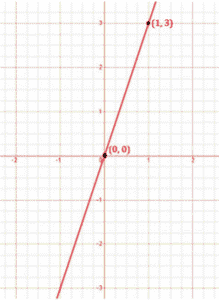# Draw the graph of the following linear equations in two variables: y = 3x

To draw the graph y=3x, we have to find the values which x and y can have, satisfying the equation.

We can check that when, x = 0, y = -2,

When x = 0,

y = 3x

y = 3×0

y = 0

When x = 1,

y = 3x

y = 3×1

y = 3

are solutions of the given equation. So, we can use the following table to draw the graph:

 x y 0 0 1 3

The points to be plotted are (0, 0) and (1, 3)